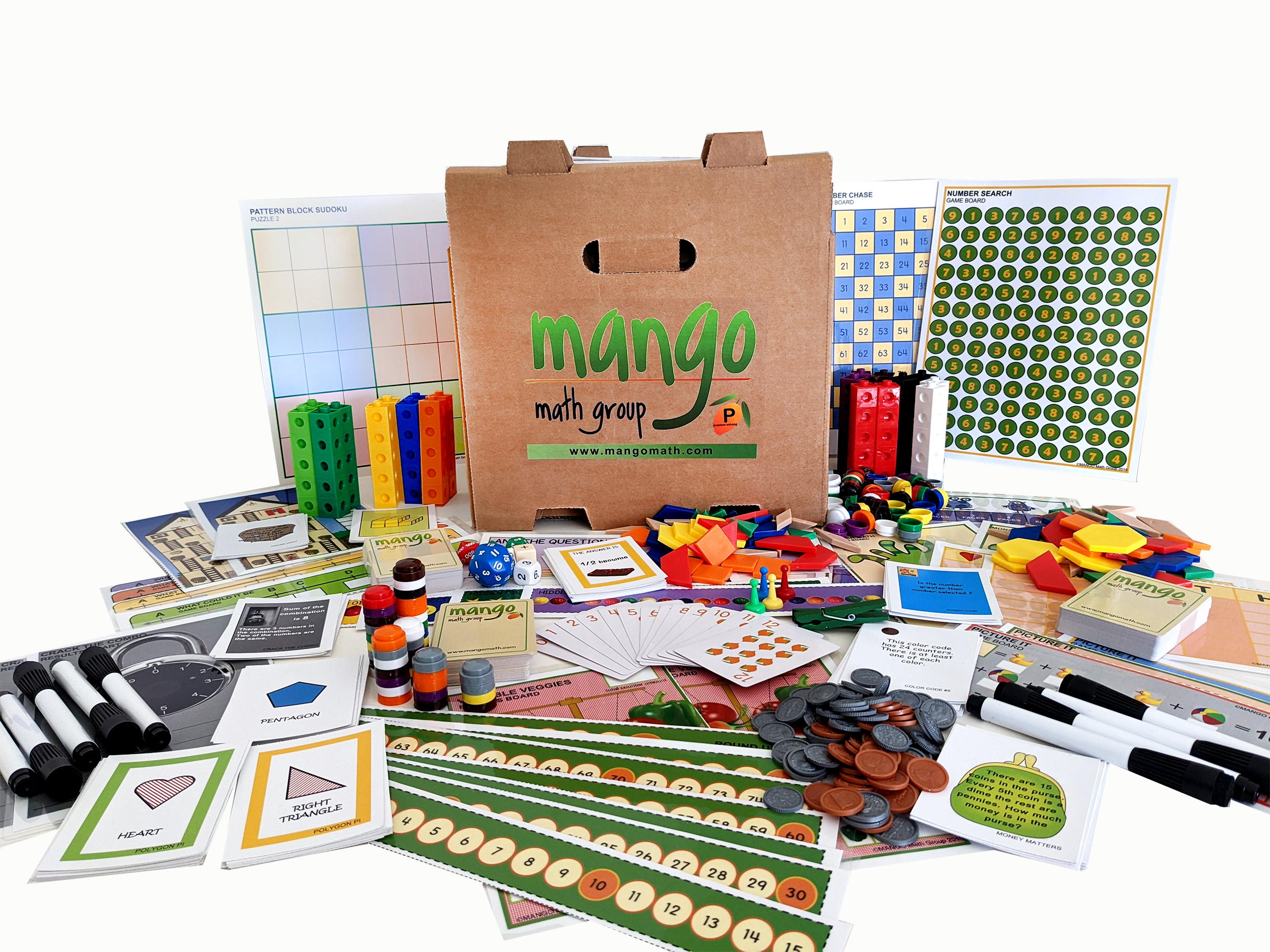#### Order Notice

As the economy opens back up, many of our suppliers are experiencing shortages of parts and raw materials, resulting in delays in shipments. As a result, we anticipate that it may take up to 2-4 weeks from the date we receive a purchase order for us to fulfill and ship any order for our products. We apologize for any inconvenience.

NEW!!

# MANGO Math K - 2 Problem Solving Kit

What better way to build math skills than through fun and easy problem-solving games and activities! MANGO Math Problem Solving Math kit is designed specifically for kindergarten, first and second grades. The kit contains 20 separate skill building math lessons.

\$ 479.00 USD##### Product Details

What better way to build math skills than through fun and easy problem-solving games and activities! MANGO Math Problem Solving Math kit is designed specifically for grades kindergarten, first and second. The kit contains 20 separate skill building math lessons.

Each individual lesson offers visual and kinetic representation of math concepts that develop strong math problem solving strategies, like:

• working backwards
• drawing a picture
• looking for patterns
• making a list
• acting it out
• guess and check
• using logic and reasoning

Every lesson is based on a game or activity that fosters curiosity, discussion and growth through cooperation, collaboration and creative thinking.

MANGO Math's Strong-Evidence Based Kits come with individually packaged, Grab-n-Go lessons that includes instructions, reusable materials pre-packaged in a resealable pouch contained in an easy to carry crate that can be used year after year, providing long term savings.

A lesson can be used by up to 4 students, set up as math centers with each grade level math kit meeting the needs of 30+ students at a time.

Each MANGO Math grade level math kit is research-based and adheres to Common Core, TEKS and NCTM math standards.

Great to use in regular classrooms, resource rooms, after school programs, STEM programs, libraries, math centers, summer camps, learning cooperatives, math clubs, community centers, and at home.

#### All the supplies below are included in this pack:

• Patten blocks
• Connecting cubes
• Plastic fruit counters
• MANGO Math playing cards
• Clothes pins
• 10s dice
• 100s dice
• 10-sided dice
• 30-sided dice
• Game pawns
• Play money
• Positive / negative dice
• Numbered dice
• Stacking counters
• Plastic resealable pouches
• Game boards
• Laminated writing boards
• Dry erase markers
• Easy to follow directions
• Curriculum guide

## Problem Solving (K – 2h grade) Lessons and Standards

Monster Math – Algebraic Thinking - Draw a Picture: Students will make a picture to help look for a pattern. Patterns will involve multiples of the same number.

Tower Total – Geometry - Act it Out: Students will create a replica of a model and determine how many total blocks are used in creating it.

Fruit Salad – Algebraic Thinking – Guess & Check: Students will solve word problems involving guessing and checking your answers.

Math Tap – Number Sense – Guess & Check: Students will use a guess and check process to determine the value on their cards.

Hidden Objects – Algebraic Thinking – Logic & Reasoning: Students will use logic and reasoning to determine a number up to 20.

Number Chase – Number Sense – Logic & Reasoning: Students will use logic and reasoning to determine the hidden number.

Pattern Block Sudoku – Logic & Reasoning: Students will use logic and reasoning to solve the puzzles.

Polygon P.I. – Geometry – Logic & Reasoning: Students will recognize rhombus, rectangles and squares as examples of quadrilaterals.

What Could They Be? – Number Sense – Logic & Reasoning: Students will use a number line to determine the value of different points on that line.

Follow Suit – Number Sense – Finding Patterns: Understand that each successive number name refers to a quantity that is one larger.

Picture It – Algebraic Thinking – Finding Patterns: Students will solve problems by determining the value of pictures by finding patterns and checking to solve equations.

Crack the Combo – Algebraic Thinking – Make a List: Using addition within 20 to solve word problems involving a situation of adding to, comparing.

I <3 Math – Algebraic Thinking – Make a Table: Students will use a table to determine a pattern to find solutions.

Number Search – Number Sense – Make it Simpler: Students will use the tactic of making problems simpler by finding “friendly” numbers.

Round Up – Number Sense – Make it Simpler: Students will round numbers to the nearest 10s to make estimating answers simpler.

Cube It – Geometry – Modeling: Students will use problem solving strategies of modeling to solve a problem.

Money Matters – Number Sense – Modeling: Students will model a problem and preform addition and subtraction within 100.  Understand value of money.

House Hunt – Number Sense – Work Backwards: Students will work backwards and attend to precision to solve a problem.

The Answer Is? – Number Sense – Working Backwards: Students will write math problem using the strategy of working backwards to create a problem that goes with the answer.

Variable Veggies – Algebraic Thinking – Working Backwards: Use addition and subtraction within 100 to solve one-and two-step word problems involving adding to, taking from, comparing with unknowns in all positions.

Width
13
in
Height
13
in
Length
19
in
Weight
20
lb